# R语言GLM广义线性模型：逻辑回归、泊松回归拟合小鼠临床试验数据（剂量和反应）示例和自测题

## 在拟合 GLM（并检查残差）之后，可以使用 z 检验一一检验估计参数的显着性，即将估计值与其标准误差进行比较。

GLM 模型拟合和分析示例

a) 我们输入数据并拟合逻辑回归模型。

``> summary(it1.lt)``

``1-pchisq(17.6,24)``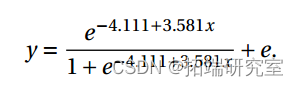``anova(fi.lgi)``
``1-pchisq(35.8-17.69,25-24)``

# 截距呢？可以删掉吗？

``````> plotx, itte(fi1log,typ"
> pot(,y``````

# R语言泊松Poisson回归模型分析案例

### 随时关注您喜欢的主题

b）我们拟合一个带有概率链接的模型。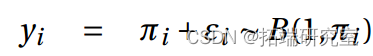``>summary()``

``>anova``
``>&nbsp;1&nbsp;-&nbsp;pchisq(35.89-17.49&nbsp;25-24)``
``>lines(x,fitte``

添加 x 时偏差的变化是显着的（P 值 =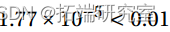）。

# 示例 2. 临床试验数据（剂量和反应）的 GLM 建模。

a) 我们输入数据，然后拟合逻辑回归模型

``>summary(it2.it)``
``1-pchisq(13.63,6)``

``>plot(x;reid(it2.it))``

``plt(fitdft2lit;reid(fi2lot))``

所以我们将 x2 添加到模型中。

``>;summary(ft2qlt)``
``>;1-pchisq(5.1,&nbsp;5)``

``plot(fitted(fit2logit),resid(.logit))``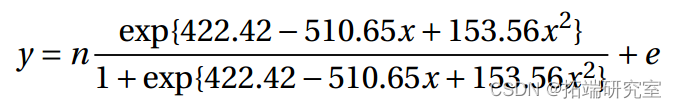# 示例 3. 艾滋病数据，泊松

a) 我们输入数据并使用默认对数链接拟合泊松回归模型。

``>smary(fit.lg)``
``>1-pchisq``

``plot(fit3esuals)``

``>summary(fi3.lg)``
``````>1-pchisq

\[1\]&nbsp;0.1279``````

16.371 的偏差（P 值为 0.1279>0.10）并不显着。拟合模型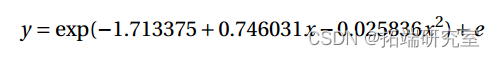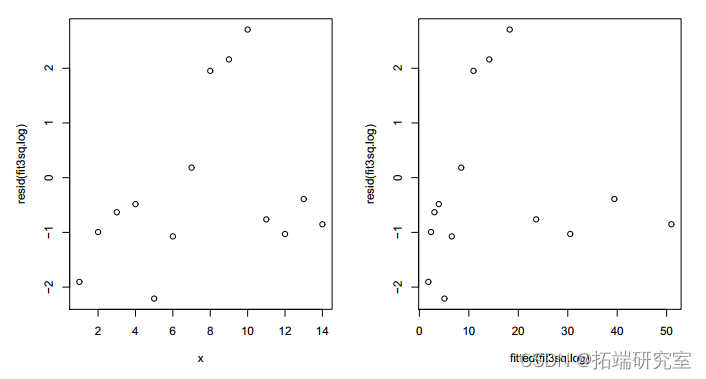``>&nbsp;summary(ft3st)``
``````> 1 - pchisq(16.9, 12)
 0.153``````

拟合模型的残差 y = (-0.27571 +0.49277x)2 + e 显示出更加随机的模式。12 df 上的偏差 16.905 略高于之前模型的 16.371（df=11），但仍然不显着（P 值=0.1532>0.10）。AIC 较小，为 73.833<75.298。因此，具有平方根链接的模型是首选。

# 自测题：

Twenty tobacco budworm moths of each sex were exposed to different doses of the insecticide trans-cypermethrin. The numbers of budworm moths killed during a 3-day exposure were as follows for each sex (male, female) and dose level in mg’s.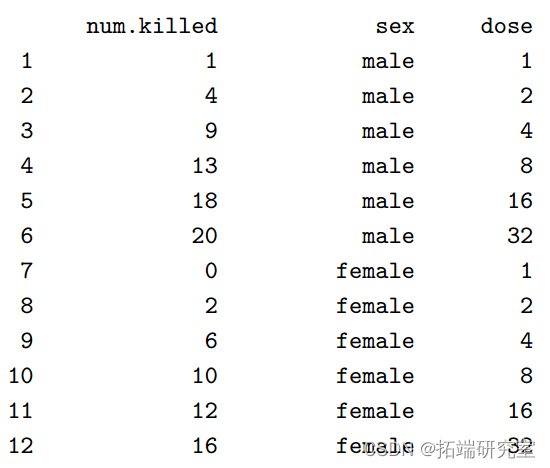Type the data into R as follows. Press Enter at the end of each line including blank lines.

``````num.killed <- scan()
1 4 9 13 18 20 0 2 6 10 12 16
sex <- scan()
0 0 0 0 0 0 1 1 1 1 1 1
dose <- scan()
1 2 4 8 16 32 1 2 4 8 16 32``````

Fit two models by doing the following.

``````ldose <- log(dose)/log(2) #convert to base-2 log dose
ldose #have a look
y <- cbind(num.killed, 20-num.killed) #add number survived
fit1 <- glm(y ~ ldose * sex, family=binomial(link=probit))
fit2 <- glm(y ~ sex + ldose, family=binomial(link=probit))``````

You may also run the following lines and refer to the chi-square distribution table

``````anova(fit1,test="Chisq")

summary(fit2)``````

1. What model is fitted in fit1? Write it formally and define all the terms.

2. How is the model in fit2 differ from that in fit1?

3. Does the model in fit1 fit the data adequately? Use deviance to answer this question.
4. Can the model in fit1 be simplified to the model in fit2? Use change in deviance to answer
this question.
5. Can sex be removed from the model in fit2? Use change in deviance to answer this ques
tion.
6. What are the maximum likelihood estimates of the parameters of the additive model? What
are their standard errors? Test the significance of each parameter using its estimate and
standard error.
7. How does the probability of a kill change with log dose and sex of the budworm moth accord

(a) Derive the survival function S(t) of a lifetime T » E xp(‚). Find ¡logS(t) and comment on it.
(b) Calculate the Kaplan-Meier estimate for each group in the following.
Treatment Group:
6,6,6,6,7,9,10,10,11,13,16,17,19,20,22,23,25,32,32,34*,35
Control Group (no treatment):
1,1,2,2,3,4,5,5,8,8,8,8,11,11,12,15,17,22,23
Note that * indicates right censored data.
(c) Use the log rank test to compare the two groups of lifetimes.

Kaizong Ye拓端研究室（TRL）的研究员。

​非常感谢您阅读本文，如需帮助请联系我们！QQ在线咨询

15121130882

0571-63341498

## 关注有关新文章的微信公众号

This will close in 0 seconds